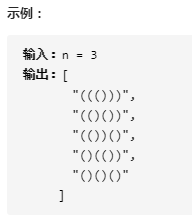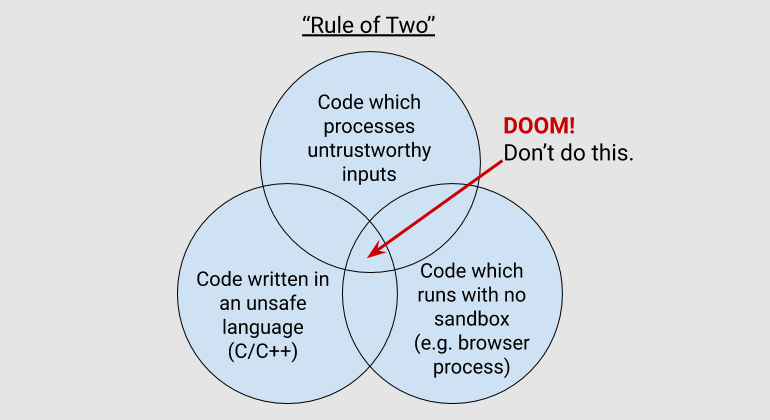# ARTS-week4Algorithm

• 题目：22. 括号生成

• 描述：数字 n 代表生成括号的对数，请你设计一个函数，用于能够生成所有可能的并且 有效的 括号组合。

• 示例:• 题解：

class Solution {public:    vector<string> generateParenthesis(int n) {        vector<string> res;        dfs(n, "", 0, 0, res);        return res;    }    void dfs(int n, string path, int nl, int nr, vector<string>& res){        if(nl>n || nr>n || nr>nl)            return;        if(nl==nr && nl == n)        {            res.push_back(path);            return;        }        dfs(n, path+"(", nl+1, nr, res);        dfs(n, path+")", nl,nr+1,res);    }};ReviewTips

• 标题：C++模板坑

• 内容：

1. 问题描述：当在.h 中声明了模板，.cpp 中定义了模板，当 main 函数去进行模板实例化的时候，在声明处找不到对应的 T 类型，自然就出问题了。

2. 解决方案：

// temp.h#ifndef _TEMP_H_#define _TEMP_H_#include <iostream>#include <vector>template <typename T>using Vec = std::vector<T>;#define PRINTFMT(x) std::cout << x << " ";template <typename T>void TestTemp(const Vec<T> &v, T target){    [=]() {        for (auto elem : v)            if (elem == target)                PRINTFMT(elem);    }();}#endif

// Temp.cpp#include "temp.h"void TestTemp(const Vec<int> &v, int target){    [=]() {        for (auto elem : v)            if (elem == target)                PRINTFMT(elem);    }();}template <typename T>void TestTemp(const Vec<T> &v, T target){    [=]() {        for (auto elem : v)            if (elem == target)                PRINTFMT(elem);    }();}

#include "temp.h"#include "temp.cpp"int main() {    std::vector<int> v{1,2,3};    int target = 2;    TestTemp<int>(v,target);    return 0;}

// temp.h#ifndef _TEMP_H_#define _TEMP_H_#include <iostream>#include <vector>template <typename T>using Vec = std::vector<T>;#define PRINTFMT(x) std::cout << x << " ";template <typename T>void TestTemp(const Vec<T> &v, T target);#include "temp.cpp"#endif

// Temp.cpp#include "temp.h"template <typename T>void TestTemp(const Vec<T> &v, T target){    [=]() {        for (auto elem : v)            if (elem == target)                PRINTFMT(elem);    }();}

#include "temp.h"int main() {    std::vector<int> v{1,2,3};    int target = 2;    TestTemp<int>(v,target);    return 0;}

Share

• 文章：变化驱动：正交设计

• 感受：

• 一个变化导致多处修改（重复）；

• 多个变化导致一处修改（多个变化方向）；

• 不依赖不必要的依赖；

• 不依赖不稳定的依赖；## 评论